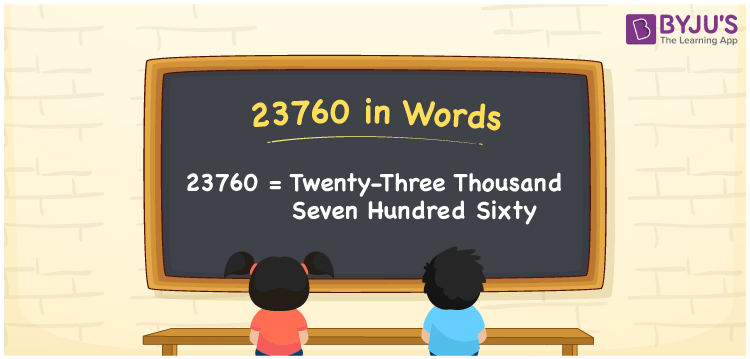# 23760 in Words

Twenty-three thousand seven hundred sixty is the number name of 23760. In mathematics, 23760 is a cardinal number, as it expresses the number of objects equivalent to twenty-three thousand seven hundred sixty. For example, Rakshita purchased a mobile worth Rs. 23760. Generally, we use the place values of the number to write the number name. Now, let us discuss the process of wiring the name 23760 in words with a complete explanation.

 23760 in Words: Twenty-three Thousand Seven Hundred Sixty. Twenty-three Thousand Seven Hundred Sixty in Numerical Form: 23760.

## 23760 in English Words## How to Write 23760 in Words?

Understand the place values of 23760, and write the number 23760 in words.

 Ten-thousands Thousands Hundreds Tens Ones 2 3 7 6 0

The expanded form of 23760 is as follows:

= 2 × Ten thousand + 3 × Thousand + 7 × Hundred + 6 × Ten + 0 × One

= 2 × 10000 + 3 × 1000 + 7 × 100 + 6 × 10 + 0 × 1

= 20000 + 3000 + 700 + 60

= 23760

= Twenty-three thousand seven hundred sixty

Hence, 23760 in words is twenty-three thousand seven hundred sixty.

23760 in words – Twenty-three thousand seven hundred sixty

Is 23760 an odd number? – No

Is 23760 an even number? – Yes

Is 23760 a perfect square number? – No

Is 23760 a perfect cube number? – No

Is 23760 a prime number? – No

Is 23760 a composite number? – Yes

## Frequently Asked Questions on 23760 in Words

Q1

### How to write 23760 in words?

23760 in words is twenty-three thousand seven hundred sixty.

Q2

### Simplify 23000 + 760, and express it in words.

Simplifying 23000 + 760, we get 23760. Hence, 23760 in words is twenty-three thousand seven hundred sixty.

Q3

### Is 23760 a composite number?

Yes, 23760 is a composite number.